# Pascal’s Triangle Shawl #2

Hooray! Hooray! Today I finished my second, prettier Pascal’s Triangle Shawl!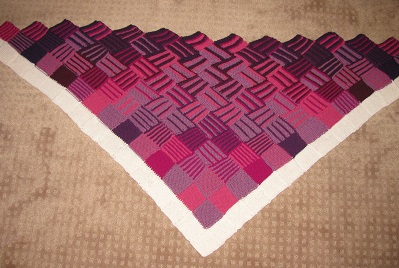Pascal’s Triangle is the triangle with 1s on the edges, where each entry is the sum of the two entries above it.

So the beginning rows work like this:

1
1 1
1 2 1
1 3 3 1
1 4 6 4 1
1 5 10 10 5 1
1 6 15 20 15 6 1
1 7 21 35 35 21 7 1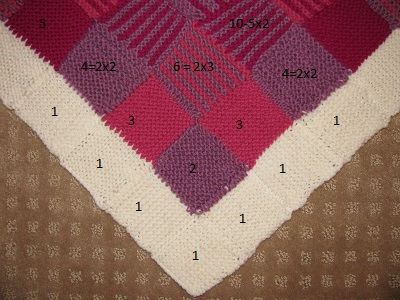Now, what I did was choose a color of yarn for each prime. Then each entry in the triangle is factored, and each number is shown by the colors of its factors.

I did the same thing with my first Pascal’s Triangle Shawl. With this one, since there are only the primes 2, 3, 5, 7, 11, and 13, I decided to use progressively darker shades of pink and purple, so the shawl would gradually get darker.

Here is a closer look at a section of the shawl: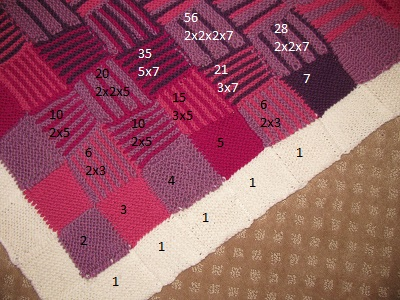This next picture shows that along the second row, we have the numbers simply in sequence.For math nuts, each row also contains the binomial coefficients, the coefficients in the expansion of
(a+b)^n

This means that the rth entry in the nth row can be calculated with the formula:
n!/(n-r)! (Counting the entries in each row as 0 through n.)

Some examples: The 2nd entry in the 5th row is (5×4)/(2×1) = 10

The 3rd entry in the 7th row is (7x6x5)/(3x2x1) = 35

Now, I factor all the numbers in my shawl, so for big numbers, it doesn’t matter what the actual number is, but the factorization is easy from the formula.

For example, the 4th entry in the 15th row is (15x14x13x12)/(4x3x2x1) = 3x5x7x13

You can see some of the bigger numbers in this picture: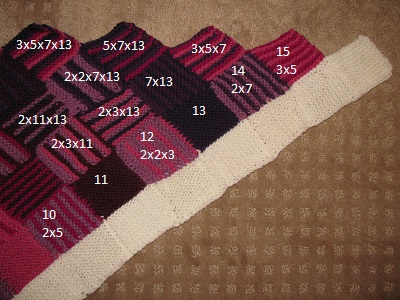Now, there are a couple of characteristics which I believe make the shawl especially beautiful.

One is that because these are the binomial coefficients, once you get to the row of a prime number, every entry in that row has the prime for a factor.

This is easier to see with the actual shawl in front of you, but here again is the big picture. You can see that once a new color starts, it goes all the way across the row.What’s more, by the distributive law, since every entry in a prime row has that prime as a factor, all the sums of those numbers will also have the prime for a factor — and we end up having inverse triangles of each color.

Here’s some more detail: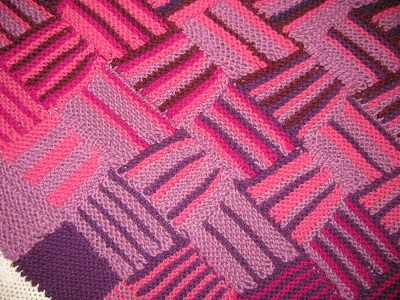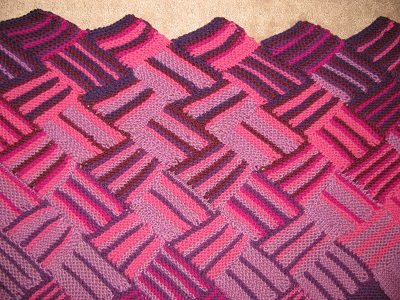Of course, the very coolest thing about it is that, even if you have no idea of the math involved, the combination is beautiful.

And that simply makes me happy.My posts on Mathematical Knitting and related topics are now gathered at Sonderknitting.

## 1 comment

1.Lorri says:

Lovely Sondy! You are very talented!